## Cost function summary

The cost function helps us figure out how to fit the most likely function to our data. For example, in model training, we have a training set (x, y), where x represents the area of ​​the house, and y represents the price of the house. We need to obtain a function h θ (x) (called a hypothesis function) through linear regression, with x As the independent variable, y is used as the dependent variable, and the function is used to predict the price under the given house area.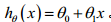The change of the parameters θ 0 and θ 1 will cause the change of the hypothesis function. The choice of parameters determines the accuracy of the straight line we get relative to the training set. The difference between the value predicted by the obtained model and the value in the training set is called the modeling error. (i.e. the blue line in the image below)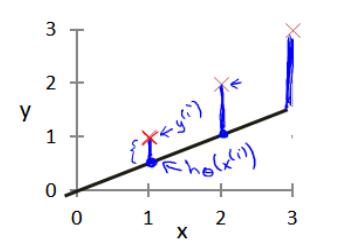Our goal is to choose the parameter that minimizes the sum of squares of the modeling error, and in the regression analysis we replace the valence function with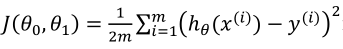, even if the value of J(θ 0 , θ 1 ) is minimal.

We draw a contour plot with the three coordinates θ 0 and θ 1 and J(θ 0, θ 1 ):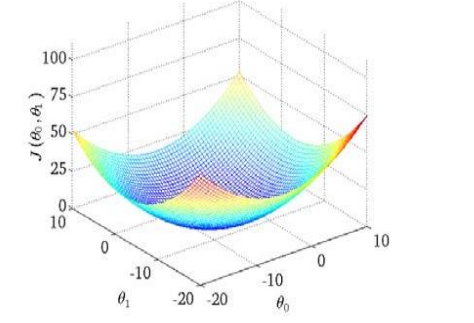It can be seen that there is a point in the three-dimensional space that minimizes the cost function J(θ 0 , θ 1 ) (ie, the lowest point in the figure)

The cost function is also called the squared error function, and is sometimes called the squared error cost function. We ask for the sum of squared errors because the squared error cost function is a reasonable choice for most problems, especially regression problems. There are other cost functions that work well too, but the squared error cost function is the most common way to solve regression problems.

There are many cost functions in machine learning. According to different problems, different cost functions are used. For example, logistic regression uses logarithmic cost function, and classification problem uses cross entropy as cost function. The commonly used loss functions are as follows: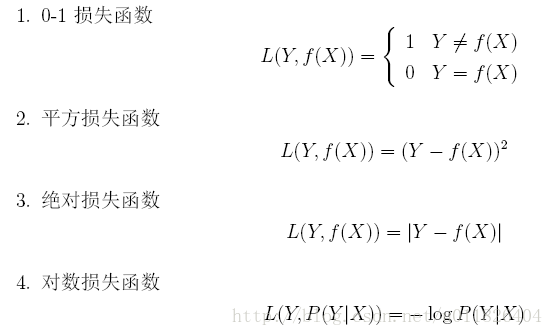So is it that the smaller the cost function, the better? The answer is No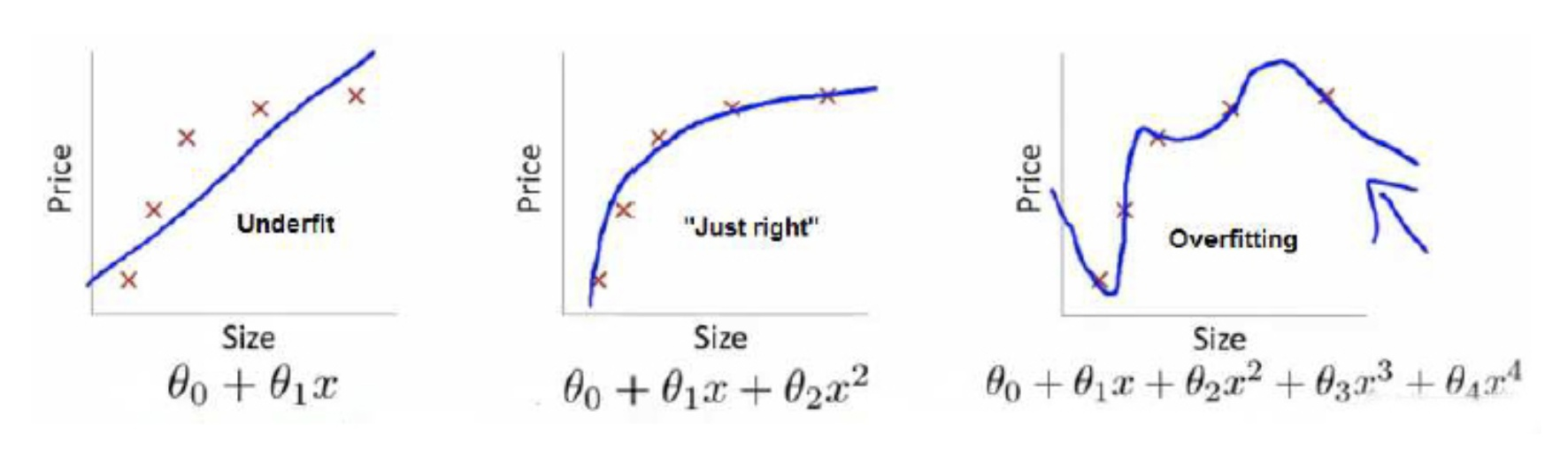The functions of the three graphs above are f 1 (x), f 2 (x), and f 3 (x) in order. We want to use these three functions to fit the real value Y respectively. From the above figure, we can see that the function f 3 (x) in Figure 3 has the best fitting effect because its cost function value is the lowest. At this time, we also need to consider empirical risk and structural risk.

So what is empirical risk and structural risk?

The average loss of f(x) with respect to the training set (X, Y) is called empirical risk, ie. "Structural risk" measures certain properties of the model, such as the complexity of the model or penalty terms, etc. The introduction of structural risk is called "regularization" in machine learning, and the commonly used ones arethenorm.

Finally, let's see why the function f 3 (x) in Figure 3 is not the best, because the function in Figure 3 overfits the training set data, resulting in overfitting, and its real prediction effect is not very good. In other words, although its fitting effect is good and the empirical risk is low, its functional complexity is good and the structural risk is high, so it is not the final obtained function. The function of Figure 2 compromises empirical risk and structural risk, so this function is an ideal function.

How to find the minimum value of the cost function? The gradient descent method will not be described here, and will be explained in detail later.

Finally, attach a simple matlab linear fitting program

x=[1:0.3:4];y=x*0.1+0.5+unifrnd(-0.5,0.5,1,11);
p=polyfit(x,y,1);
x1=linspace(min(x),max(x));
y1=polyval(p,x1);
plot(x,y,'*',x1,y1);


### Guess you like

Origin http://43.154.161.224:23101/article/api/json?id=325261837&siteId=291194637
Recommended
Ranking
Daily